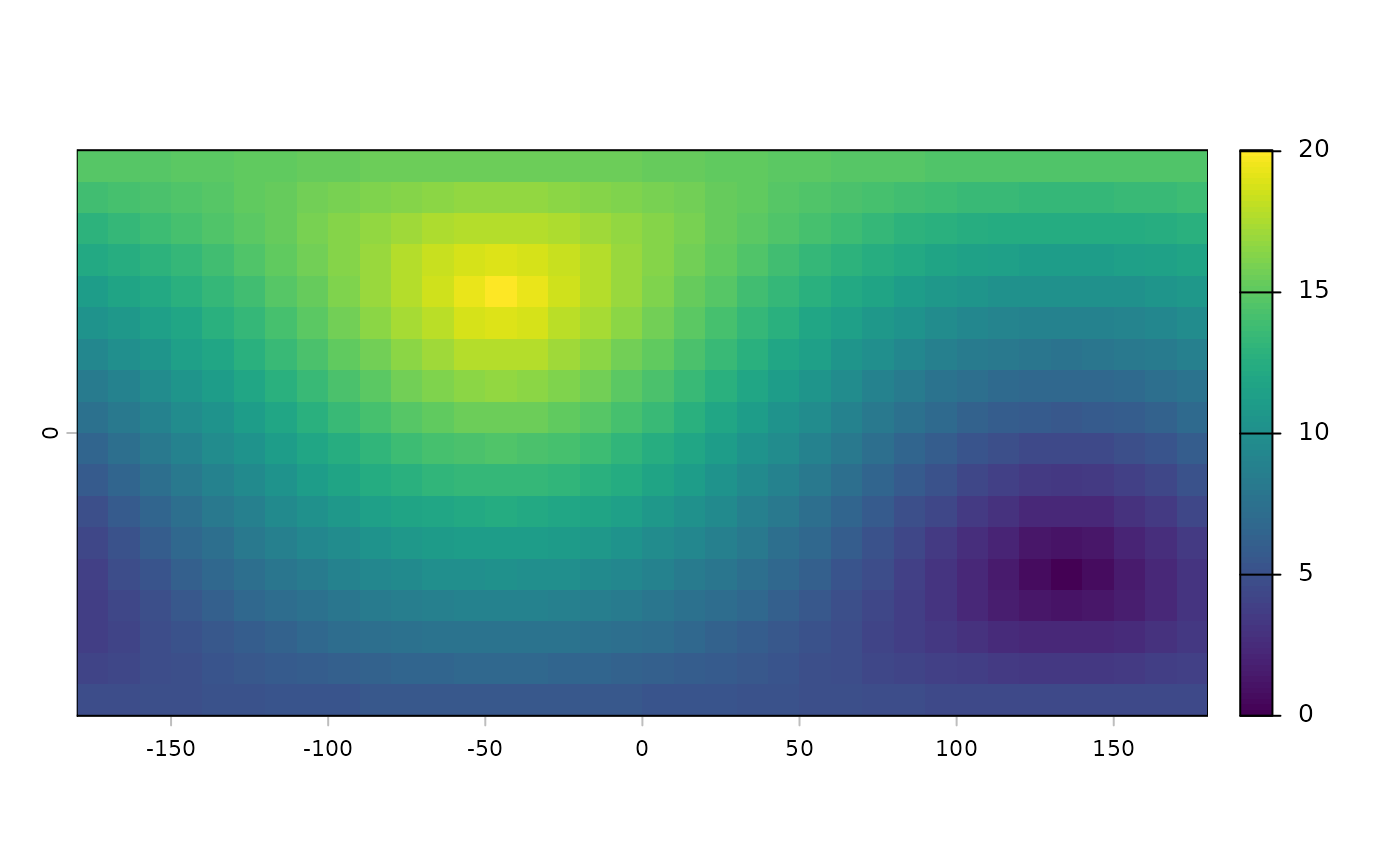If x is a SpatRaster:

If y is missing this method computes the distance, for all cells that are NA in SpatRaster x to the nearest cell that is not NA (or other values, see arguments "target" and "exclude".

If y is a numeric value, the cells with that value are ignored. That is, distance to or from these cells is not computed (only if grid=FALSE).

If y is a SpatVector, the distance to that SpatVector is computed for all cells. For lines and polygons this is done after rasterization; and only the overlapping areas of the vector and raster are considered (for now).

The distance is always expressed in meter if the coordinate reference system is longitude/latitude, and in map units otherwise. Map units are typically meter, but inspect crs(x) if in doubt.

Results are more precise, sometimes much more precise, when using longitude/latitude rather than a planar coordinate reference system, as these distort distance.

If x is a SpatVector:

If y is missing, a distance matrix between all object in x is computed. An distance matrix object of class "dist" is returned.

If y is a SpatVector the geographic distance between all objects is computed (and a matrix is returned). If both sets have the same number of points, and pairwise=TRUE, the distance between each pair of objects is computed, and a vector is returned.

The distance is always expressed in meter, except when the coordinate reference system is longitude/latitude AND one of the SpatVector(s) consists of lines or polygons. In that case the distance is in degrees, and thus not very useful (this will be fixed soon). Otherwise, results are more precise, sometimes much more precise, when using longitude/latitude rather than a planar coordinate reference system, as these distort distance.

If x is a matrix:

x should consist of two columns, the first with "x" (or longitude) and the second with "y" coordinates (or latitude). If y is a also a matrix, the distance between each points in x and all points in y is computed, unless pairwise=TRUE

If y is missing, the distance between each points in x with all other points in x is computed, unless sequential=TRUE

# S4 method for SpatRaster,missing
distance(x, y, target=NA, exclude=NULL, unit="m", haversine=TRUE, filename="", ...)

# S4 method for SpatRaster,SpatVector
distance(x, y, unit="m", rasterize=FALSE, haversine=TRUE, filename="", ...)

# S4 method for SpatVector,ANY
distance(x, y, sequential=FALSE, pairs=FALSE, symmetrical=TRUE, unit="m")

# S4 method for SpatVector,SpatVector
distance(x, y, pairwise=FALSE, unit="m")

# S4 method for matrix,matrix
distance(x, y, lonlat, pairwise=FALSE)

# S4 method for matrix,missing
distance(x, y, lonlat, sequential=FALSE, pairs=FALSE, symmetrical=TRUE)

## Arguments

x

SpatRaster, SpatVector, or two-column matrix with coordinates (x,y) or (lon,lat)

y

missing, numeric, SpatVector, or two-column matrix

target

numeric. The value of the cells for which distances to cells that are not NA should be computed

exclude

numeric. The value of the cells that should not be considered for computing distances

unit

character. Can be either "m" or "km"

haversine

logical. Use the haversine formula for lon/lat data use the haversine formula? If FALSE, the more precise but slower method of Karney (2003) is used

rasterize

logical. If TRUE distance is computed from the cells covered by the geometries after rasterization. This can be much faster in some cases

filename

character. Output filename

...

additional arguments for writing files as in writeRaster

sequential

logical. If TRUE, the distance between sequential geometries is returned

pairwise

logical. If TRUE and if x and y have the same size (number of rows), the pairwise distances are returned instead of the distances between all elements

lonlat

logical. If TRUE the coordinates are interpreted as angular (longitude/latitude). If FALSE they are interpreted as planar

pairs

logical. If TRUE a "from", "to", "distance" matrix is returned

symmetrical

logical. If TRUE and pairs=TRUE, the distance between a pair is only included once. The distance between geometry 1 and 3 is included, but the (same) distance between 3 and 1 is not

## Value

SpatRaster or numeric or matrix or distance matrix (object of class "dist")

## Note

The distance unit is in meters.

A distance matrix can be coerced into a matrix with as.matrix

## Examples

#lonlat
r <- rast(ncols=36, nrows=18, crs="+proj=longlat +datum=WGS84")
r <- 1
d <- distance(r)
#>
|---------|---------|---------|---------|
=========================================

plot(d / 100000)#planar
rr <- rast(ncols=36, nrows=18, crs="+proj=utm +zone=1 +datum=WGS84")
rr <- 1
d <- distance(rr)

rr[3:10, 3:10] <- 99
e <- distance(rr, exclude=99)

p1 <- vect(rbind(c(0,0), c(90,30), c(-90,-30)), crs="+proj=longlat +datum=WGS84")
dp <- distance(r, p1)
#>
|---------|---------|---------|---------|
=========================================

d <- distance(p1)
d
#>          1        2
#> 2 10014577
#> 3 10014577 20003931
as.matrix(d)
#>          1        2        3
#> 1        0 10014577 10014577
#> 2 10014577        0 20003931
#> 3 10014577 20003931        0

p2 <- vect(rbind(c(30,-30), c(25,40), c(-9,-3)), crs="+proj=longlat +datum=WGS84")
dd <- distance(p1, p2)
dd
#>          [,1]     [,2]     [,3]
#> [1,]  4596223  5104507  1054933
#> [2,]  9192445  5905838 11048088
#> [3,] 10825924 14117180  8981037
pd <- distance(p1, p2, pairwise=TRUE)
pd
#>  4596223 5905838 8981037
pd == diag(dd)
#>  TRUE TRUE TRUE

# polygons, lines
crs <- "+proj=utm +zone=1"
p1 <- vect("POLYGON ((0 0, 8 0, 8 9, 0 9, 0 0))", crs=crs)
p2 <- vect("POLYGON ((5 6, 15 6, 15 15, 5 15, 5 6))", crs=crs)
p3 <- vect("POLYGON ((2 12, 3 12, 3 13, 2 13, 2 12))", crs=crs)
p <- rbind(p1, p2, p3)
L1 <- vect("LINESTRING(1 11, 4 6, 10 6)", crs=crs)
L2 <- vect("LINESTRING(8 14, 12 10)", crs=crs)
L3 <- vect("LINESTRING(1 8, 12 14)", crs=crs)
lns <- rbind(L1, L2, L3)
pts <- vect(cbind(c(7,10,10), c(3,5,6)), crs=crs)

distance(p1,p3)
#>      [,1]
#> [1,]    3
distance(p)
#>   1 2
#> 2 0
#> 3 3 2
distance(p,pts)
#>          [,1]     [,2]     [,3]
#> [1,] 0.000000 2.000000 2.000000
#> [2,] 3.000000 1.000000 0.000000
#> [3,] 9.848858 9.899495 9.219544
distance(p,lns)
#>          [,1]     [,2]     [,3]
#> [1,] 0.000000 3.535534 0.000000
#> [2,] 0.000000 0.000000 0.000000
#> [3,] 1.414214 5.099020 2.553878
distance(pts,lns)
#>      [,1]     [,2]     [,3]
#> [1,]    3 8.602325 7.262591
#> [2,]    1 5.385165 6.943356
#> [3,]    0 4.472136 6.065460